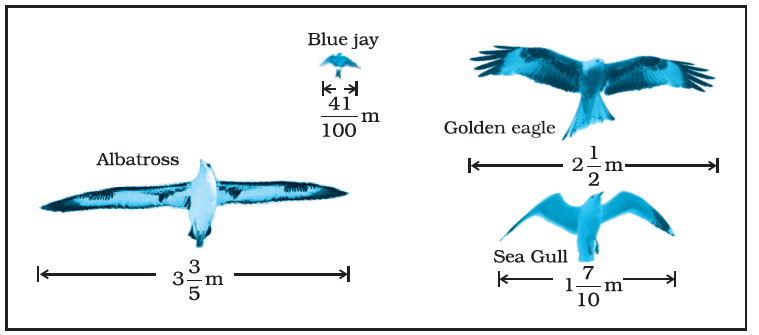# The diagram shows the wingspans of different species of birds. Use the diagram to answer the question given below: (a) How much longer is the wingspan of an Albatross than the wingspan of a Sea gull? (b) How much longer is the wingspan of a Golden eagle than the wingspan of a Blue jay?Solution

(a)

We have to find out the difference of wingspan of an Albatross and wingspan of a Sea gull.

Length of wingspan of an Albatross = $$3\frac{3}{5}$$ = 18/5 m

Length of wingspan of a Sea gull =$$1\frac{7}{10}$$ = 17/10 m

Difference of both( sea gull and albatross) = (18/5) – (17/10)

= (36 – 17)/ 10

= 19/10 m

∴The wingspan of an Albatross is 19/10 m longer than the wingspan of a Sea gull.

(b)

We have to find out the difference of wingspan of a Golden eagle and wingspan of a Blue jay.

Length of wingspan of a Golden eagle = $$2\frac{1}{2}$$= $$\frac{5}{2}$$ m

Length of wingspan of a Blue jay = $$\frac{41}{100}$$ m

Difference of both = latex]\frac{5}{2}\)– $$\frac{41}{100}$$

=$$\frac{250-41}{100}$$

=$$\frac{209}{100}$$

∴The wingspan of a Golden eagle is 209/100 m longer than the wingspan of a Blue jay.(0)(0)